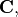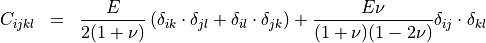# Stiffness¶

Type: 4-Tensor, or section [v_1, …, v_81] -/- optional

Defines stiffness tensoralso called elasticity tensor. Units are pascals,, which corresponds to force per unit area.

This stiffness tensor relates the strainof a body to the stresses within the body. In the used linear form this relation is given by:whereis the initial strain of the body, i.e., the strain for which the body is free of stresses.

When the Poisson ratio(see PoissonRatio) and the Young modulus(see YoungModulus) are set, a stiffness tensor is implicitly defined by the following expression when not otherwise specified: